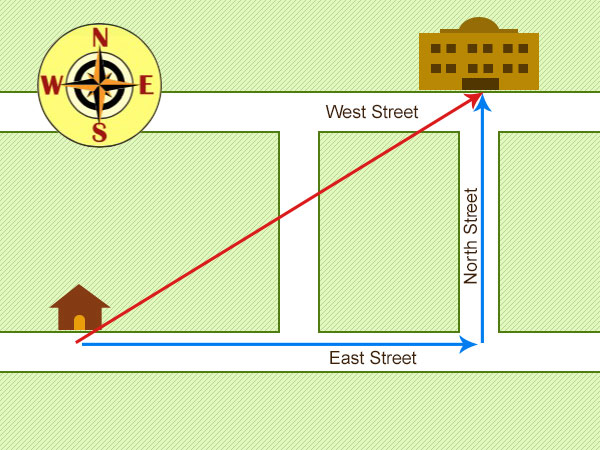## Distance and Displacement

### Distance

Distance is a measure of how far an object moves. It is a scalar quantity and only requires the magnitude (size) along with an appropriate unit to describe it.

If someone was asked how far their house was from school and they answered, “2” it would not be a complete answer. The follow up question would be, “2 what?” If they then answer, “2km” only then is a full description of the magnitude of the distance from their house to school is given. Thus, distance is a scalar quantity meaning it can be fully described by the magnitude and appropriate unit.

### Displacement

Displacement is the distance travelled in a particular direction from a specified point. It includes both the distance an object moves, measured in a straight line from the start point to the finish point and the direction of that straight line. It is a vector quantity as it has both magnitude (size) and direction.

Following on from the example given in the distance section, if the person was asked, “How do you get from your house to school?” and they replied, “walk 2km” the answer would be incomplete. The follow up question would be, “In which direction?” Only by answering with, “Walk 2km due east” is a complete description given.

## Distance and Displacement Examples

### Example 2Hannah leaves her house to go to the library. She walks 400m east along East Street and then 300m north along North Street to reach the library.

Distance:
The distance is how far Hannah has moved (blue lines).

Distance = 400m + 300m = 700m

Displacement:
Displacement is the distance travelled in a particular direction from a specified point (red line).

It can be measured using a ruler if the map is to scale. In this case it can be calculated using Pythagoras’s theorem for a right angled triangle.